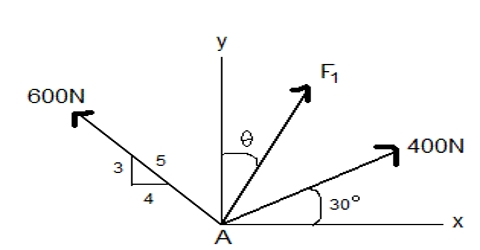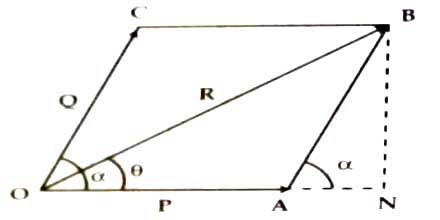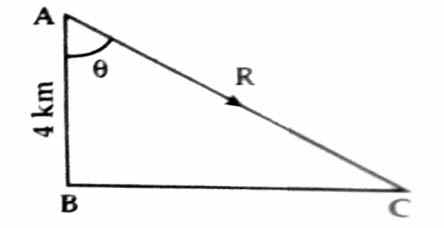# Determination of Magnitude of Resultant

Determination of magnitude of the resultant:

Suppose the magnitude of the resultant is R and AOC = α is an acute angle (Figure). Now from point B let us draw normal BN on the extended line of OA which intersects the line OA at N.

Now, AB and OC are parallel

so, AOC = BAN = α

Again in, ∆OBN, ONB = 900

so, OB2 = ON2 + BN2 = (OA + AN)2 + BN2

= OA2 + 2OA.AN + AN2 + BN2

But, AB2 = AN2 + BN2 [here, ∆BNA is a right angle triangle]

or, OC2 = AN2 + BN2 [AB = OC]Now, from trigonometry,

AN = AB cos α = OC cos α

so, OB2 = OA2 + OC2 + 2OA.AB cos α

or, OB2 = OA2 + OC2 + 2OA.OC cos α

or, R2 = P2 + Q2 + 2PQ cos α

then, R = √(P2 + Q2 + 2PQ cos α) … … … (i)

Direction of the Resultant:

Suppose the resultant R makes an angle θ with P; i.e., AOB = θ

so, in the right angle ∆OBN,

tan θ = BN/ON = BN/(OA+AN)

= AB sinα / (OA+AB Cosα) (here, sinα = BN/AB and cosα = AN/AB)

so, tanθ = Q sinα / (P+Qcosθ) … … … (ii)

Hence, from equation (i) and (ii), we get R and θ recpectively;

Vectors AB and BC represent 4 km and 10 km displacements respectively along the North and the East. According to the law of triangle the resultant of the displacement vector AC is drawn.If the angle between AB and AC is θ and the magnitude of the resultant vector is R, then;

R = √(102+42) = √116 = 10.78 km

and tan θ = 10/4 = 2.5

so, θ = 68.20